Processing ......FreeComputerBooks.com Links to Free Computer, Mathematics, Technical Books all over the World

Graph Theory Lessons
🌠 Top Free Machine Learning Books - 100% Free or Open Source!
• Title Graph Theory Lessons
• Authors Christopher P. Mawata
• Publisher: Math Cove (2018); University of Tennessee at Chattanooga (2004)
• Paperback: N/A
• eBook HTML
• Language: English
• ISBN-10: N/A
• ISBN-13: N/A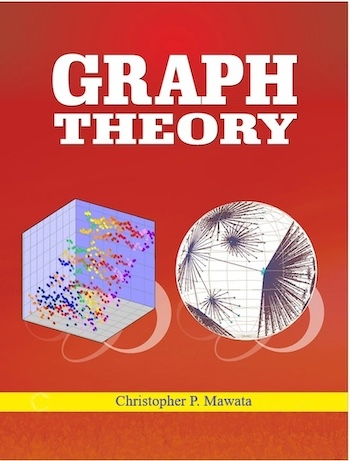Book Description

This comprehensive text covers the important elementary topics of graph theory and its applications. In addition, he presents a large variety of proofs designed to strengthen mathematical techniques and offers challenging opportunities to have fun with mathematics.

It utilizes a java software, Petersen, written by Christopher P. Mawata, which can draw, edit and manipulate simple graphs, examine properties of the graphs, and demonstrate them using computer animation. The software can display information about a graph like the number of vertices and their degrees, the adjacency matrix, the number of components, and articulation points.

It can also find complements of graphs, line graphs, find the chromatic number of a graph, check if a graph is bipartite, check if two graphs are isomorphic or if one graph is a subgraph of another and find the dual graph of a planar graph in many cases. Petersen also demonstrates Euler and Hamilton circuits, searches, and algorithms for finding minimum spanning trees.

• N/A
Reviews, Ratings, and Recommendations: Related Book Categories: Read and Download Links:Similar Books:
•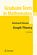Graph Theory (Reinhard Diestel)

This book covers the core material of the subject with concise yet reliably complete proofs, while offering glimpses of more advanced methods in each field by one or two deeper results, again with proofs given in full detail.

•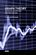Graph Theory - Advanced Algorithms and Applications

Not only will the methods and explanations help you to understand more about graph theory, but you will find it joyful to discover ways that you can apply graph theory in your applications or scientific research.

•Graph Theory and Complex Networks (Maarten van Steen)

This book aims to explain the basics of graph theory that are needed at an introductory level for students in computer or information sciences. It also aims to provide an introduction to the modern field of network science.

•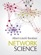Network Science (Albert-László Barabási)

Illustrated throughout in full colour, this pioneering textbook, spanning a wide range of topics from physics to computer science, engineering, economics and the social sciences, introduces network science to an interdisciplinary audience.

•Probability on Trees and Networks (Russell Lyons, et al.)

This book is concerned with certain aspects of discrete probability on infinite graphs that are currently in vigorous development. Of course, finite graphs are analyzed as well, but usually with the aim of understanding infinite graphs and networks.

•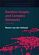Random Graphs and Complex Networks (Remco van der Hofstad)

This rigorous introduction to network science presents Random Graphs as models for real-world networks. Such networks have distinctive empirical properties and a wealth of new models have emerged to capture them.

•Graph Algorithms: Practical Examples in Apache Spark and Neo4j

This book is a practical guide to getting started with graph algorithms for developers and data scientists who have experience using Apache Spark or Neo4j. You'll walk through hands-on examples that show you how to use graph algorithms in Apache Spark/Neo4j.

•A Survey of Statistical Network Models (Anna Goldenberg, et al.)

This book aims to provide the reader with an entry point to the voluminous literature on statistical network modeling. It guides the reader through the development of key stochastic network models, touches upon a number of examples and commonalities.

•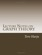Lecture Notes on Graph Theory (Tero Harju)

These are introductory lecture notes on graph theory. It offers undergraduates a remarkably student-friendly introduction to graph theory and takes an engaging approach that emphasizes graph theory's history.

•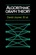Algorithmic Graph Theory (David Joyner, et al)

This is an introductory book on algorithmic graph theory. Theory and algorithms are illustrated using the Sage open source mathematics software. It's especially suitable for computer scientists and mathematicians interested in computational complexity.

•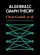Explorations in Algebraic Graph Theory (Chris Godsil, et al.)

This book aims to express properties of graphs in algebraic terms, then to deduce theorems about them. It tackles the applications of linear algebra and matrix theory to the study of graphs; algebraic constructions such as adjacency matrix, using the Sage.

•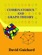An Introduction to Combinatorics and Graph Theory

This book walks the reader through the classic parts of Combinatorics and graph theory, while also discussing some recent progress in the area: on the one hand, providing material that will help students learn the basic techniques.

•Graph Databases: New Opportunities for Connected Data

This book provides a practical foundation for those who want to apply Graph Database to real-world business solutions. You'll learn why graph database are useful, where they're applicable, and how to design and implement solutions that use them.

Book Categories
 :All CategoriesTop Free BooksRecent BooksMiscellaneous BooksComputer EngineeringComputer LanguagesComputer ScienceData Science/DatabasesJava and Java EE (J2EE)Linux and UnixMathematicsMicrosoft and .NETMobile ComputingNetworking and CommunicationsSoftware EngineeringSpecial TopicsWeb Programming
Other Categories